In :
%matplotlib inline
from numpy import linspace, log, exp
from numpy.random import normal
from scipy.stats import chisqprob
from lmfit import Model
import seaborn as sns
sns.set_style('ticks')
sns.set_context('notebook', font_scale=1)


# The likelihood ratio test: Python implementation¶

For two models, one nested in the other (meaning that the nested model estimated parameters are a subset of the nesting model), the test statistic $D$ is (based on this):

$$\Lambda = \Big( \Big(\frac{\sum{(X_i - \hat{X_i}(\theta_1))^2}}{\sum{(X_i - \hat{X_i}(\theta_0))^2}}\Big)^{n/2} \Big) \\ D = -2 log \Lambda \\ lim_{n \to \infty} D \sim \chi^2_{df=\Delta}$$

where $\Lambda$ is the likelihood ratio, $D$ is the statistic, $X_{i}$ are the data points, $\hat{X_i}(\theta)$ is the model prediction with parameters $\theta$, $\theta_i$ is the parameters estimation for model $i$, $n$ is the number of data points and $\Delta$ is the difference in number of parameters between the models.

The python implementation below compares between two lmfit.ModelFit objects. These are the results of fitting models to the same data set using the lmfit package.

The function compares between model fit m0 and m1 and assumes that m0 is nested in m1, meaning that the set of varying parameters of m0 is a subset of the varying parameters of m1. The property chisqr of the ModelFit objects is the sum of the square of the residuals of the fit. ndata is the number of data points. nvarys is the number of varying parameters.

In :
def lrtest(m0, m1, alfa=0.05):
"""Perform a likelihood ratio test on two nested models.

Input:

m0 is nested in m1.
alfa is the test significance level (default 5%).

Output:

prefer_m1 - a boolean, should we prefer m1 over m0.
pval - the test p-value
D - the test statistic
ddf - the number of degrees of freedom
"""
n0 = m0.ndata
k0 = m0.nvarys
chisqr0 = m0.chisqr
assert chisqr0 > 0
n1 = m1.ndata
assert n0 == n1
k1 = m1.nvarys
chisqr1 = m1.chisqr
assert chisqr1 > 0
Lambda = (m1.chisqr / m0.chisqr)**(n0 / 2.)
D = -2 * log( Lambda )
assert D > 0
ddf = k1 - k0
assert ddf > 0
pval = chisqprob(D, ddf)
prefer_m1 = pval < alfa
return prefer_m1, pval, D, ddf


## Test on a simple model¶

We test the function on data generated from the equation:

$$y = b + e^{-a t} + N(0, \sigma^2)$$

where $a$ and $b$ are the parameters, $t$ is the variable, and $N$ is the normal distribution.

We fit a nesting model model_fit1 (the alternative hypothesis of the test). This model estimates both $a$ and $b$. We also fit a nested model model_fit0 (the nul hypothesis of the test) in which either $a$ or $b$ is fixed at an initial value. We than plot both model fits, print the estimated parameters, the test statistic and the p-value of the test.

In :
# test lrt
def lrtest_test(a, b, a_init, b_init, a_vary, b_vary, sig=0.1, N=100, alfa=0.05):
assert a_vary or b_vary
assert not(a_vary and b_vary)

t = linspace(0,1,N)
f = lambda t,a,b: b + exp(-a*t)
data = f(t,a,b) + normal(0, sig, N)
fig, ax = subplots(1,2,sharex=True,sharey=True,figsize=(15,5))
print "real:", a,b
model = Model(f)
params = model.make_params(a=a_init, b=b_init)

two_var_fit = model.fit(data, t=t, params=params)
two_var_fit.plot_fit(ax=ax)
ax.set_title("Two variables")
#model_fit1.plot_residuals(ax)
print "Two variables:",two_var_fit.best_values

params['a'].set(vary=a_vary)
params['b'].set(vary=b_vary)
one_var_fit = model.fit(data, t=t, params=params)
one_var_fit.plot_fit(ax=ax)
#model_fit0.plot_residuals(ax)
ax.set_title("One variable")
print "One variable:",one_var_fit.best_values

prefer_m1,pval,D,ddf = lrtest(one_var_fit, two_var_fit, alfa)
print "pval=%.2g, D=%.2g, ddf=%d" % (pval, D, ddf)
print "Decision with significance level %.2g:" % alfa,
if prefer_m1:
print "Two variable model"
else:
print "One variable model"
return t,data

In :
lrtest_test(1,1,1,1,False,True);

real: 1 1
Two variables: {'a': 0.86775531514445625, 'b': 0.97560666838937937}
One variable: {'a': 1, 'b': 1.0118956348766281}
pval=0.19, D=1.7, ddf=1
Decision with significance level 0.05: One variable model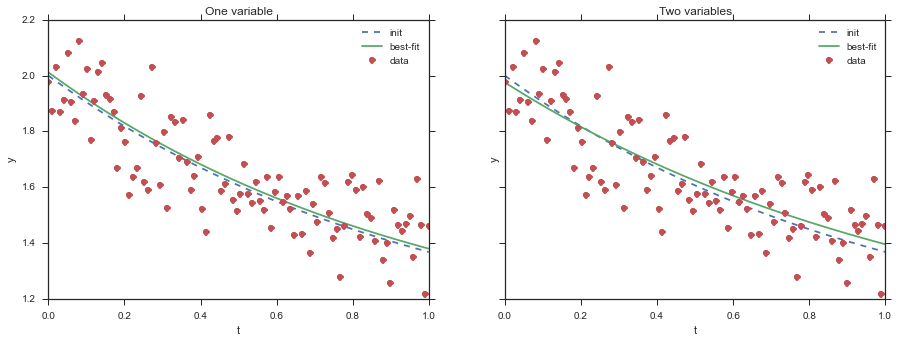In :
lrtest_test(1,1,1,1,True,False);

real: 1 1
Two variables: {'a': 0.88198851749015894, 'b': 0.95415310004056564}
One variable: {'a': 1.0278836663608819, 'b': 1}
pval=0.042, D=4.1, ddf=1
Decision with significance level 0.05: Two variable model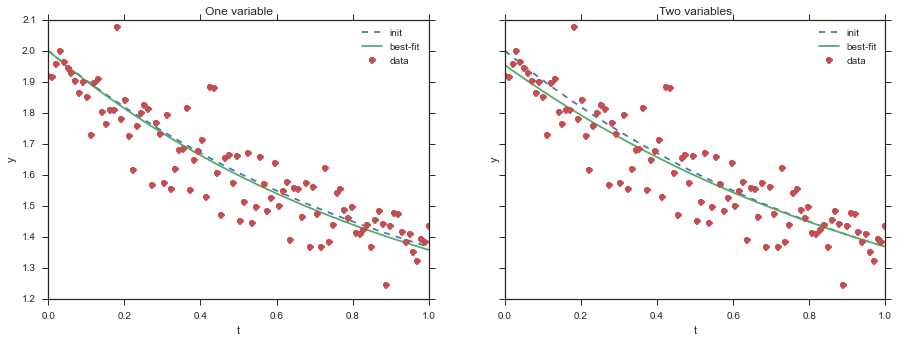In :
lrtest_test(1,1,0,0,True,False);

real: 1 1
Two variables: {'a': 1.0842648241878579, 'b': 1.0287763184769507}
One variable: {'a': -0.5729794466048922, 'b': 0}
pval=1.2e-72, D=3.2e+02, ddf=1
Decision with significance level 0.05: Two variable model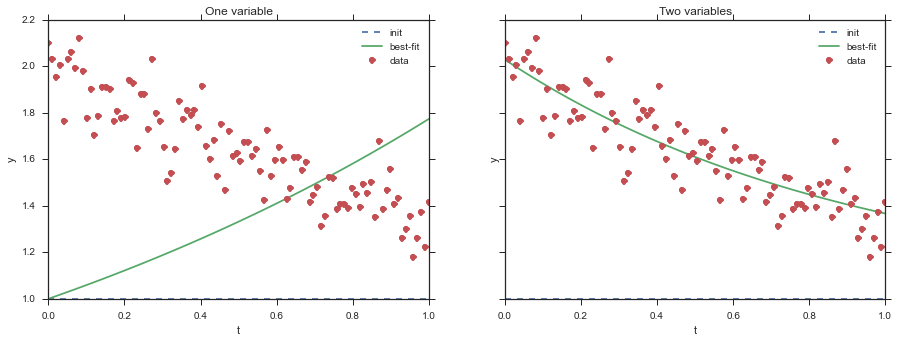In :
lrtest_test(1,1,0,0,False,True);

real: 1 1
Two variables: {'a': 0.9452008165850978, 'b': 0.98442967528266856}
One variable: {'a': 0, 'b': 0.63175416158525943}
pval=5.3e-35, D=1.5e+02, ddf=1
Decision with significance level 0.05: Two variable model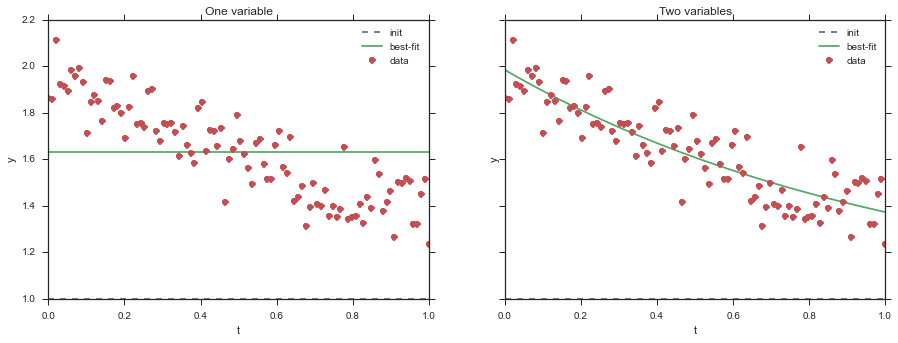In :
lrtest_test(1,1,0,1,False,True);

real: 1 1
Two variables: {'a': 0.98872695387898135, 'b': 0.98522534209144352}
One variable: {'a': 0, 'b': 0.62084932431698936}
pval=7.3e-35, D=1.5e+02, ddf=1
Decision with significance level 0.05: Two variable model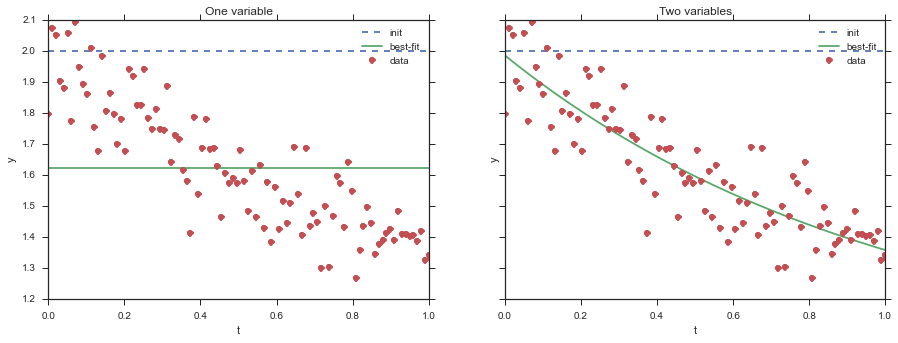In :
lrtest_test(1,1,0,1,True,False);

real: 1 1
Two variables: {'a': 1.0224399232088139, 'b': 0.99340603684830542}
One variable: {'a': 1.0446412443876858, 'b': 1}
pval=0.8, D=0.065, ddf=1
Decision with significance level 0.05: One variable model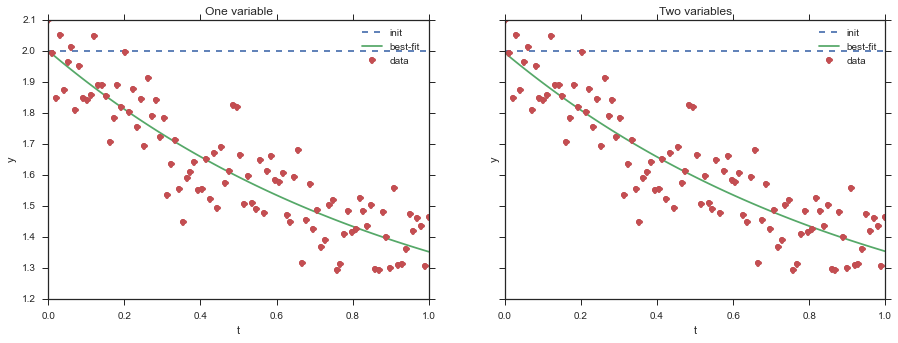In :
lrtest_test(1,1,1,0,True,False);

real: 1 1
Two variables: {'a': 0.87089207465018115, 'b': 0.96616302997657855}
One variable: {'a': -0.58724547087449808, 'b': 0}
pval=5e-74, D=3.3e+02, ddf=1
Decision with significance level 0.05: Two variable model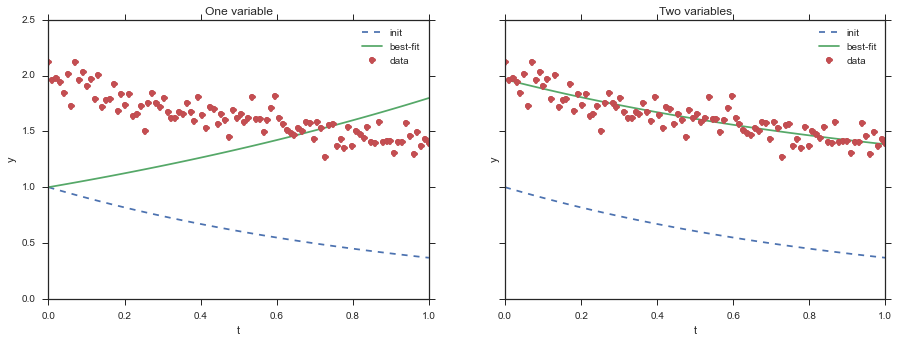In :
lrtest_test(1,1,1,0,False,True);

real: 1 1
Two variables: {'a': 1.0285746095656456, 'b': 1.0174174562149918}
One variable: {'a': 1, 'b': 1.0099552907882328}
pval=0.77, D=0.086, ddf=1
Decision with significance level 0.05: One variable model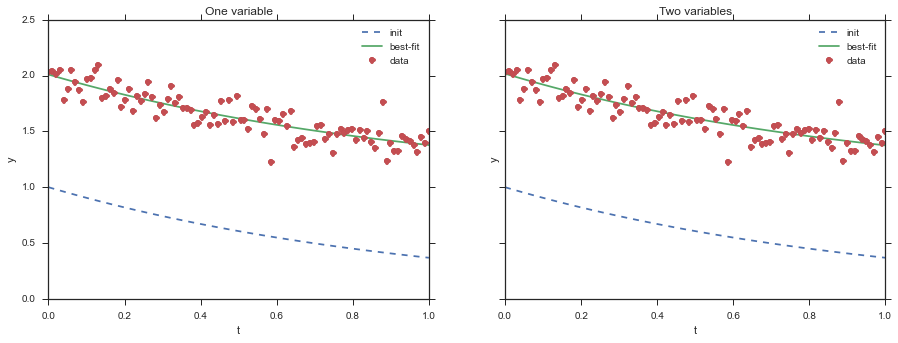In :
lrtest_test(1,1,2,1,False,True);

real: 1 1
Two variables: {'a': 1.0888695144064144, 'b': 1.0317987702059201}
One variable: {'a': 2, 'b': 1.2079536785181528}
pval=3.3e-07, D=26, ddf=1
Decision with significance level 0.05: Two variable model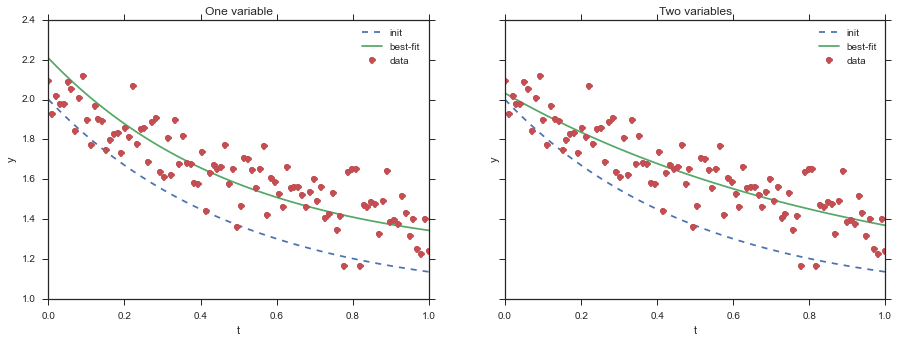## Compare with R¶

Here we compare the Python implementation with the R implementation - lmtest::lrtest. The data is generated from a quadratic polynomial:

$$y = at^2 + bt + c + N(0,\sigma^2)$$

and we fit two models - the nested model is a linear function and the nesting model is a quadratic polynomial.

### Note¶

In order to get this to work you will need to make sure the lmtest package is installed (don't install via RStudio - use regular command line R) and that the R_HOME and R_USER environment variable are set to where R is running from the command line and where the packages are installed to when running from the command line.

In :
%load_ext rpy2.ipython

The rpy2.ipython extension is already loaded. To reload it, use:

In :
!echo %R_HOME%
!echo %R_USER%

C:/Progra~1/R/R-3.0.3
C:\Users\yoavram\R

In :
for _ in range(10):
N = 100
sig = 0.1
a,b,c = 0.1,1,1
t = linspace(0,1,N)
f = lambda t,a,b,c: c + b*t + a*t**2
data = f(t,a,b,c) + normal(0, sig, N)
model = Model(f)
params = model.make_params(a=0, b=0, c=0)

model_fit1 = model.fit(data, t=t, params=params)

params['a'].set(vary=False)
model_fit0 = model.fit(data, t=t, params=params)

prefer_m1,pval,D,ddf = lrtest(model_fit0, model_fit1)
print "py",
print pval

print "R ",
%Rpush t data
%R library(lmtest)
%R lm1=lm(data~t)
%R lm2=lm(data~t+I(t^2))
%R pvalue = lrtest(lm2,lm1)[2,5]
%Rpull pvalue
print pvalue

py 0.0179882275173
R  0.0179882275173
py 0.537531566876
R  0.537531566876
py 0.269018479047
R  0.269018479047
py 0.495471455839
R  0.495471455839
py 0.546745762151
R  0.546745762151
py 0.662389288421
R  0.662389288421
py 0.534273698101
R  0.534273698101
py 0.0422040728343
R  0.0422040728343
py 0.633756809221
R  0.633756809221
py 0.203276605564
R  0.203276605564